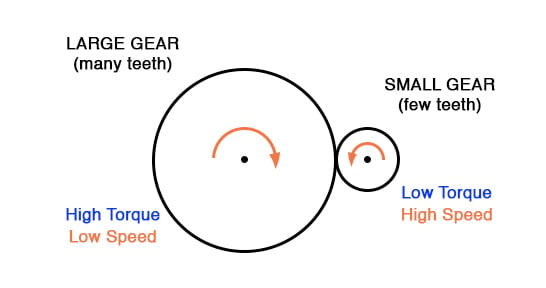## What is a step-up transformer?

A transformer designed to increase voltage from primary to secondary is called a step-up transformer.

# Step-up and Step-down Transformers

## Chapter 9 - Transformers

So far, we’ve observed simulations of transformers where the primary and secondary windings were of identical inductance, giving approximately equal voltage and current levels in both circuits. Equality of voltage and current between the primary and secondary sides of a transformer, however, is not the norm for all transformers.

If the inductances of the two windings are not equal, something interesting happens:

transformer
v1 1 0 ac 10 sin
rbogus1 1 2 1e-12
rbogus2 5 0 9e12
l1 2 0 10000
l2 3 5 100
k l1 l2 0.999
vi1 3 4 ac 0
.ac lin 1 60 60
.print ac v(2,0) i(v1)
.print ac v(3,5) i(vi1)
.end

freq          v(2)        i(v1)
6.000E+01     1.000E+01   9.975E-05    Primary winding

freq          v(3,5)      i(vi1)
6.000E+01     9.962E-01   9.962E-04    Secondary winding


Notice how the secondary voltage is approximately ten times less than the primary voltage (0.9962 volts compared to 10 volts), while the secondary current is approximately ten times greater (0.9962 mA compared to 0.09975 mA).

What we have here is a device that steps the voltage down by a factor of ten and current up by a factor of ten: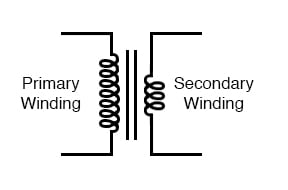Turns ratio of 10:1 yields 10:1 primary: secondary voltage ratio and 1:10 primary: secondary current ratio.

#### What are Step-up and Step-down Transformers?

This is a very useful device, indeed. With it, we can easily multiply or divide voltage and current in AC circuits. Indeed, the transformer has made the long-distance transmission of electric power a practical reality, as AC voltage can be “stepped up” and current “stepped down” for reduced wire resistance power losses along power lines connecting generating stations with loads.

At either end (both the generator and at the loads), voltage levels are reduced by transformers for safer operation and less expensive equipment.

A transformer that increases the voltage from primary to secondary (more secondary winding turns than primary winding turns) is called a step-up transformer.

Conversely, a transformer designed to do just the opposite is called a step-down transformer.

Let’s re-examine a photograph shown in the previous section: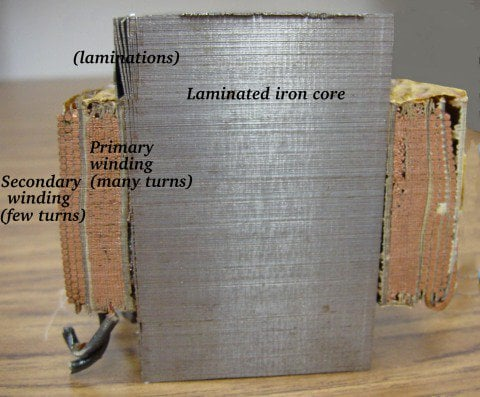Transformer cross-section showing primary and secondary windings is a few inches tall (approximately 10 cm).

This is a step-down transformer, as evidenced by the high turn count of the primary winding and the low turn count of the secondary. As a step-down unit, this transformer converts high-voltage, low-current power into low-voltage, high-current power.

The larger-gauge wire used in the secondary winding is necessary due to the increase in current. The primary winding, which doesn’t have to conduct as much current, may be made of smaller-gauge wire.

#### Reversibility of Transformer Operation

In case you were wondering, it is possible to operate either of these transformer types backward (powering the secondary winding with an AC source and letting the primary winding power a load) to perform the opposite function: a step-up can function as a step-down and visa-Versa.

However, as we saw in the first section of this chapter, efficient operation of a transformer requires that the individual winding inductances be engineered for specific operating ranges of voltage and current, so if a transformer is to be used “backward” like this it must be employed within the original design parameters of voltage and current for each winding, lest it prove to be inefficient (or lest it be damaged by excessive voltage or current!).

#### Transformer Construction Labels

Transformers are often constructed in such a way that it is not obvious which wires lead to the primary winding and which lead to the secondary. One convention used in the electric power industry to help alleviate confusion is the use of “H” designations for the higher-voltage winding (the primary winding in a step-down unit; the secondary winding in a step-up) and “X” designations for the lower-voltage winding.

Therefore, a simple power transformer will have wires labeled “H1”, “H2”, “X1”, and “X2”. It is usually significant to the numbering of the wires (H1 versus H2, etc.), which we’ll explore a little later in this chapter.

#### Practical Significance of Step-Up and Step-Down Transformers

The fact that voltage and current get “stepped” in opposite directions (one up, the other down) makes perfect sense when you recall that power is equal to voltage times current, and realize that transformers cannot produce power, only convert it.

Any device that could output more power than it took in would violate the Law of Energy Conservation in physics, namely that energy cannot be created or destroyed, only converted. As with the first transformer example we looked at, power transfer efficiency is very good from the primary to the secondary sides of the device.

The practical significance of this is made more apparent when an alternative is considered: before the advent of efficient transformers, voltage/current level conversion could only be achieved through the use of motor/generator sets.

A drawing of a motor/generator set reveals the basic principle involved: (Figure below)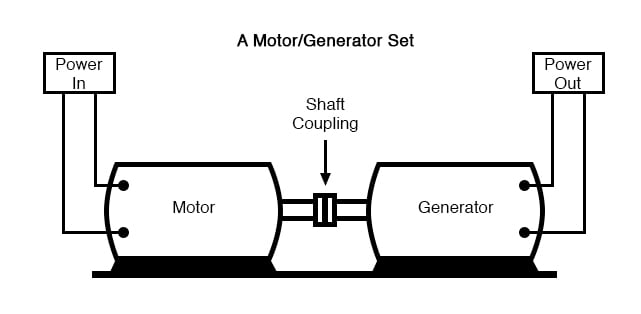=

Motor generator illustrates the basic principle of the transformer.

In such a machine, a motor is mechanically coupled to a generator, the generator designed to produce the desired levels of voltage and current at the rotating speed of the motor.

While both motors and generators are fairly efficient devices, the use of both in this fashion compounds their inefficiencies so that the overall efficiency is in the range of 90% or less. Furthermore, because motor/generator sets obviously require moving parts, mechanical wear and balance are factors influencing both service life and performance.

Transformers, on the other hand, are able to convert levels of AC voltage and current at very high efficiencies with no moving parts, making possible the widespread distribution and use of electric power we take for granted.

In all fairness, it should be noted that motor/generator sets have not necessarily been obsoleted by transformers for all applications.

While transformers are clearly superior over motor/generator sets for AC voltage and current level conversion, they cannot convert one frequency of AC power to another, or (by themselves) convert DC to AC or visa-versa.

Motor/generator sets can do all these things with relative simplicity, albeit with the limitations of efficiency and mechanical factors already described.

Motor/generator sets also have the unique property of kinetic energy storage: that is, if the motor’s power supply is momentarily interrupted for any reason, its angular momentum (the inertia of that rotating mass) will maintain rotation of the generator for a short duration, thus isolating any loads powered by the generator from “glitches” in the main power system.

#### Analysis of Step-up and Step-down Transformer Operation

Looking closely at the numbers in the SPICE analysis, we should see a correspondence between the transformer’s ratio and the two inductances. Notice how the primary inductor (l1) has 100 times more inductance than the secondary inductor (10000 H versus 100 H), and that the measured voltage step-down ratio was 10 to 1.

The winding with more inductance will have a higher voltage and less current than the other.

Since the two inductors are wound around the same core material in the transformer (for the most efficient magnetic coupling between the two), the parameters affecting inductance for the two coils are equal except for the number of turns in each coil.

If we take another look at our inductance formula, we see that inductance is proportional to the square of the number of coil turns: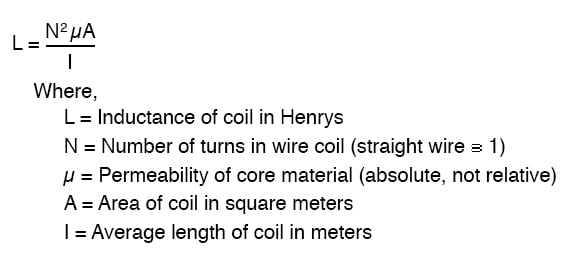So, it should be apparent that our two inductors in the last SPICE transformer example circuit—with inductance ratios of 100:1—should have coil turn ratios of 10:1, because of 10 squared equals 100.

This works out to be the same ratio we found between primary and secondary voltages and currents (10:1), so we can say as a rule that the voltage and current transformation ratio is equal to the ratio of winding turns between primary and secondary.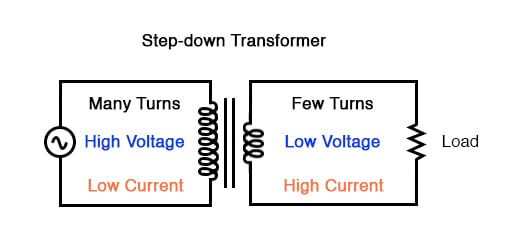Step-down transformer: (many turns :few turns).

The step-up/step-down effect of coil turn ratios in a transformer is analogous to gear tooth ratios in mechanical gear systems, transforming values of speed and torque in much the same way:Torque reducing gear train steps torque down, while stepping speed up.

Step-up and step-down transformers for power distribution purposes can be gigantic in proportion to the power transformers previously shown, some units standing as tall as a home. The following photograph shows a substation transformer standing about twelve feet tall: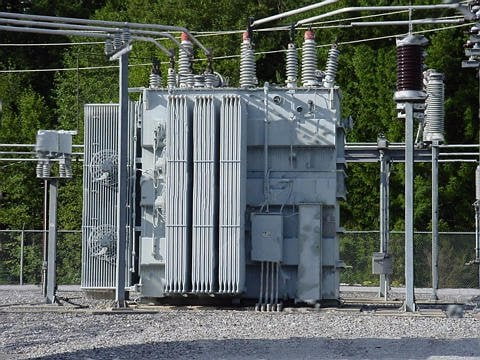Substation transformer.

REVIEW:

• Transformers “step up” or “step down” voltage according to the ratios of primary to secondary wire turns.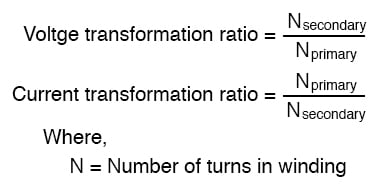• A transformer designed to increase the voltage from primary to secondary is called a step-up transformer. A transformer designed to reduce the voltage from primary to secondary is called a step-down transformer.
• The transformation ratio of a transformer will be equal to the square root of its primary to secondary inductance (L) ratio.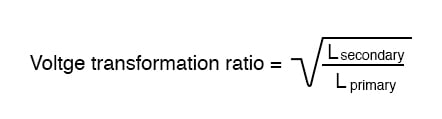RELATED WORKSHEETS:

• Share
Published under the terms and conditions of the Design Science License